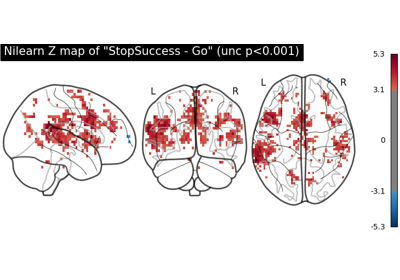Note

This page is a reference documentation. It only explains the function signature, and not how to use it. Please refer to the user guide for the big picture.

# nilearn.plotting.plot_img_comparison#

nilearn.plotting.plot_img_comparison(ref_imgs, src_imgs, masker, plot_hist=True, log=True, ref_label='image set 1', src_label='image set 2', output_dir=None, axes=None)[source]#

Creates plots to compare two lists of images and measure correlation.

The first plot displays linear correlation between voxel values. The second plot superimposes histograms to compare values distribution.

Parameters:
ref_imgsnifti_like

Reference images.

src_imgsnifti_like

Source images.

Mask to be used on data.

plot_histBoolean, optional

If True then histograms of each img in ref_imgs will be plotted along-side the histogram of the corresponding image in src_imgs. Default=True.

logBoolean, optional

Passed to plt.hist. Default=True.

ref_labelstr, optional

Name of reference images. Default=’image set 1’.

src_labelstr, optional

Name of source images. Default=’image set 2’.

output_dirstring, optional

Directory where plotted figures will be stored.

axeslist of two matplotlib Axes objects, optional

Can receive a list of the form [ax1, ax2] to render the plots. By default new axes will be created.

Returns:
corrsnumpy.ndarray

Pearson correlation between the images.

## Examples using `nilearn.plotting.plot_img_comparison`#First level analysis of a complete BIDS dataset from openneuro

First level analysis of a complete BIDS dataset from openneuro